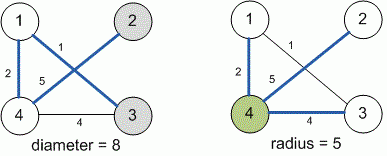Competitions

# Diameter of the graph

The connected weighted and undirected graph is given.

Consider the pair of vertices, for which the distance between them is maximum among all pairs of vertices. Such distance is called the graph diameter. The eccentricity of the vertex v is the maximal distance from the vertex v to all other vertices of the graph. The radius of the graph is minimal eccentricity of the vertices.

Find the diameter and the radius of the graph.

#### Input

The first line contains the number of graph vertices n (1n100). Each of the next n lines contains n numbers - the adjacency matrix of the graph, where -1 means the absence of the edge between the vertices, and any nonnegative integer means the edge with the given weight. The main diagonal of the matrix contains zeros; the edge weights do not exceed 1000.

#### Output

Print two numbers: the diameter and the radius of the graph.Time limit 1 second
Memory limit 128 MiB
Input example #1
4
0 -1 1 2
-1 0 -1 5
1 -1 0 4
2 5 4 0
Output example #1
8
5